# Cuboctahedron

Cuboctahedron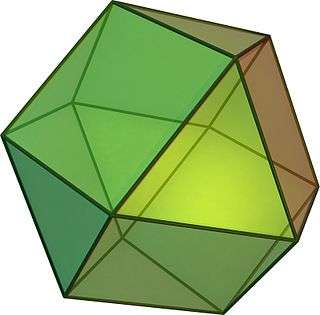TypeArchimedean solid
Uniform polyhedron
ElementsF = 14, E = 24, V = 12 (χ = 2)
Faces by sides8{3}+6{4}
Conway notationaC
aaT
Schläfli symbolsr{4,3} orrr{3,3} ort1{4,3} or t0,2{3,3}
Wythoff symbol2 | 3 4
3 3 | 2
Coxeter diagramoror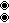Symmetry groupOh, B3, [4,3], (*432), order 48
Td, [3,3], (*332), order 24
Rotation groupO, [4,3]+, (432), order 24
Dihedral Angle125.26°
arcsec(−3)
ReferencesU07, C19, W11
PropertiesSemiregular convex quasiregular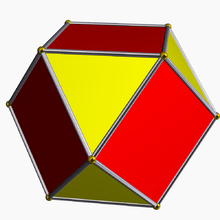Colored faces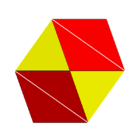3.4.3.4
(Vertex figure)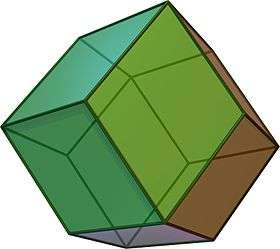Rhombic dodecahedron
(dual polyhedron)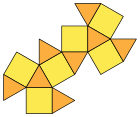Net

In geometry, a cuboctahedron is a polyhedron with 8 triangular faces and 6 square faces. A cuboctahedron has 12 identical vertices, with 2 triangles and 2 squares meeting at each, and 24 identical edges, each separating a triangle from a square. As such, it is a quasiregular polyhedron, i.e. an Archimedean solid that is not only vertex-transitive but also edge-transitive.

Its dual polyhedron is the rhombic dodecahedron.

The cuboctahedron was probably known to Plato: Heron's Definitiones quotes Archimedes as saying that Plato knew of a solid made of 8 triangles and 6 squares.

## Other names

• Heptaparallelohedron (Buckminster Fuller)
• Fuller applied the name "Dymaxion" to this shape, used in an early version of the Dymaxion map. He also called it the "Vector Equilibrium". He called a cuboctahedron consisting of rigid struts connected by flexible vertices a "jitterbug" (this shape can be deformed into an octahedron, tetrahedron or icosahedron by collapsing its square sides).
• With Oh symmetry, order 48, it is a rectified cube or rectified octahedron (Norman Johnson)
• With Td symmetry, order 24, it is a cantellated tetrahedron or rhombitetratetrahedron.
• With D3d symmetry, order 12, it is a triangular gyrobicupola.

## Area and volume

The area A and the volume V of the cuboctahedron of edge length a are:## Orthogonal projections

The cuboctahedron has four special orthogonal projections, centered on a vertex, an edge, and the two types of faces, triangular and square. The last two correspond to the B2 and A2 Coxeter planes. The skew projections show a square and hexagon passing through the center of the cuboctahedron.

Cuboctahedron (orthogonal projections)
Square
Face
Triangular
Face
Vertex Edge Skew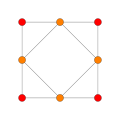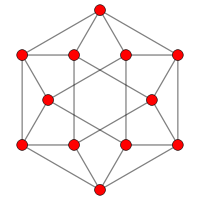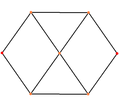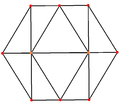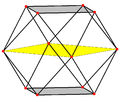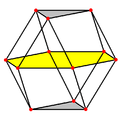Rhombic dodecahedron (Dual polyhedron)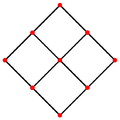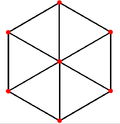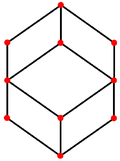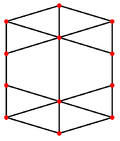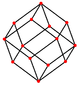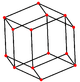## Spherical tiling

The cuboctahedron can also be represented as a spherical tiling, and projected onto the plane via a stereographic projection. This projection is conformal, preserving angles but not areas or lengths. Straight lines on the sphere are projected as circular arcs on the plane.

 orthographic projection Stereographic projections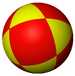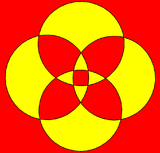square-centered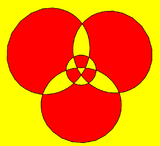triangle-centered

## Cartesian coordinates

The Cartesian coordinates for the vertices of a cuboctahedron (of edge length 2) centered at the origin are:

(±1,±1,0)
(±1,0,±1)
(0,±1,±1)

An alternate set of coordinates can be made in 4-space, as 12 permutations of:

(0,1,1,2)

This construction exists as one of 16 orthant facets of the cantellated 16-cell.

### Root vectors

The cuboctahedron's 12 vertices can represent the root vectors of the simple Lie group A3. With the addition of 6 vertices of the octahedron, these vertices represent the 18 root vectors of the simple Lie group B3.

## Dissection

The cuboctahedron can be dissected into two triangular cupolas by a common hexagon passing through the center of the cuboctahedron. If these two triangular cupolas are twisted so triangles and squares line up, Johnson solid J27, the triangular orthobicupola, is created.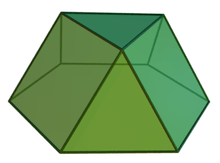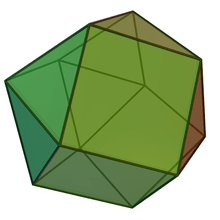The cuboctahedron can also be dissected into 6 square pyramids and 8 tetrahedra meeting at a central point. This dissection is expressed in the alternated cubic honeycomb where pairs of square pyramids are combined into octahedra.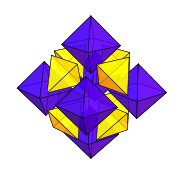## Geometric relations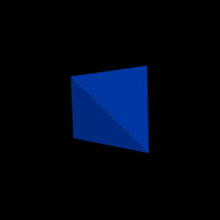Progression between a tetrahedron, expanded into a cuboctahedron, and reverse expanded into the dual tetrahedron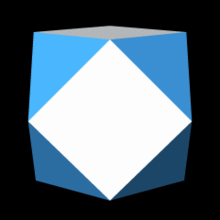Progressions between an octahedron, pseudoicosahedron, and cuboctahedron

A cuboctahedron can be obtained by taking an appropriate cross section of a four-dimensional 16-cell.

A cuboctahedron has octahedral symmetry. Its first stellation is the compound of a cube and its dual octahedron, with the vertices of the cuboctahedron located at the midpoints of the edges of either.

The cuboctahedron is a rectified cube and also a rectified octahedron.

It is also a cantellated tetrahedron. With this construction it is given the Wythoff symbol: 3 3 | 2.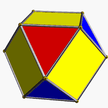A skew cantellation of the tetrahedron produces a solid with faces parallel to those of the cuboctahedron, namely eight triangles of two sizes, and six rectangles. While its edges are unequal, this solid remains vertex-uniform: the solid has the full tetrahedral symmetry group and its vertices are equivalent under that group.

The edges of a cuboctahedron form four regular hexagons. If the cuboctahedron is cut in the plane of one of these hexagons, each half is a triangular cupola, one of the Johnson solids; the cuboctahedron itself thus can also be called a triangular gyrobicupola, the simplest of a series (other than the gyrobifastigium or "digonal gyrobicupola"). If the halves are put back together with a twist, so that triangles meet triangles and squares meet squares, the result is another Johnson solid, the triangular orthobicupola, also called an anticuboctahedron.

Both triangular bicupolae are important in sphere packing. The distance from the solid's center to its vertices is equal to its edge length. Each central sphere can have up to twelve neighbors, and in a face-centered cubic lattice these take the positions of a cuboctahedron's vertices. In a hexagonal close-packed lattice they correspond to the corners of the triangular orthobicupola. In both cases the central sphere takes the position of the solid's center.

Cuboctahedra appear as cells in three of the convex uniform honeycombs and in nine of the convex uniform 4-polytopes.

The volume of the cuboctahedron is 5/6 of that of the enclosing cube and 5/8 of that of the enclosing octahedron.

### Vertex arrangement

The cuboctahedron shares its edges and vertex arrangement with two nonconvex uniform polyhedra: the cubohemioctahedron (having the square faces in common) and the octahemioctahedron (having the triangular faces in common). It also serves as a cantellated tetrahedron, as being a rectified tetratetrahedron.Cuboctahedron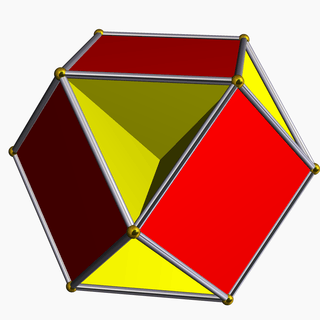Cubohemioctahedron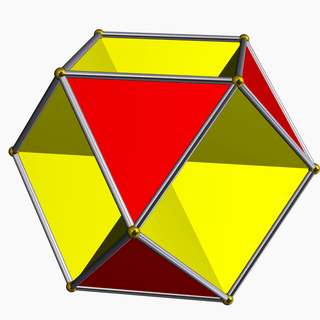Octahemioctahedron

The cuboctahedron 2-covers the tetrahemihexahedron, which accordingly has the same abstract vertex figure (two triangles and two squares: 3.4.3.4) and half the vertices, edges, and faces. (The actual vertex figure of the tetrahemihexahedron is 3.4.3/2.4, with the a/2 factor due to the cross.)Cuboctahedron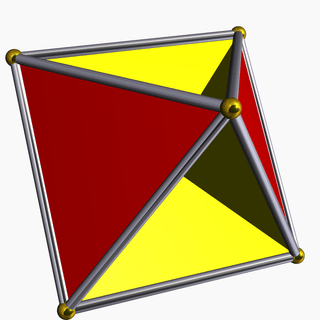Tetrahemihexahedron

The cuboctahedron is one of a family of uniform polyhedra related to the cube and regular octahedron.

The cuboctahedron also has tetrahedral symmetry with two colors of triangles.

The cuboctahedron exists in a sequence of symmetries of quasiregular polyhedra and tilings with vertex configurations (3.n)2, progressing from tilings of the sphere to the Euclidean plane and into the hyperbolic plane. With orbifold notation symmetry of *n32 all of these tilings are wythoff construction within a fundamental domain of symmetry, with generator points at the right angle corner of the domain.

This polyhedron is topologically related as a part of sequence of cantellated polyhedra with vertex figure (3.4.n.4), and continues as tilings of the hyperbolic plane. These vertex-transitive figures have (*n32) reflectional symmetry.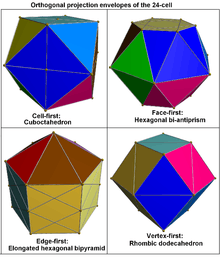Orthogonal projections of 24-cell

The cuboctahedron can be decomposed into a regular octahedron and eight irregular but equal octahedra in the shape of the convex hull of a cube with two opposite vertices removed. This decomposition of the cuboctahedron corresponds with the cell-first parallel projection of the 24-cell into three dimensions. Under this projection, the cuboctahedron forms the projection envelope, which can be decomposed into six square faces, a regular octahedron, and eight irregular octahedra. These elements correspond with the images of six of the octahedral cells in the 24-cell, the nearest and farthest cells from the 4D viewpoint, and the remaining eight pairs of cells, respectively.

## Cultural occurrences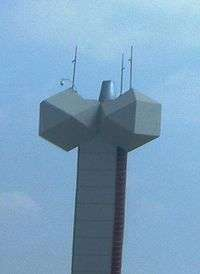Two cuboctahedra on a chimney in Israel.
• In the Star Trek episode "By Any Other Name", aliens seize the Enterprise by transforming crew members into inanimate cuboctahedra.
• The "Geo Twister" fidget toy is a flexible cuboctahedron.
• The Coriolis space stations in the computer game series Elite are cuboctahedron-shaped.
• Vesak Kuudu, traditional lanterns made in Sri Lanka annually to celebrate Vesak Poya day, are usually cuboctahedral.

## Cuboctahedral graph

Cuboctahedral graph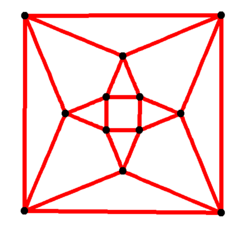4-fold symmetry
Vertices 12
Edges 24
Automorphisms 48
Properties Quartic graph, Hamiltonian, regular

In the mathematical field of graph theory, a cuboctahedral graph is the graph of vertices and edges of the cuboctahedron, one of the Archimedean solids. It has 12 vertices and 24 edges, and is a quartic Archimedean graph.6-fold symmetry

1. Heath, Thomas L. (1931), A manual of Greek mathematics, Clarendon, p. 176
2. Vector Equilibrium: R. Buckminster Fuller
3. Richter, David A., Two Models of the Real Projective Plane
4. Coxeter, H. S. M. (1973), Regular Polytopes (3rd ed.), Dover, Chapter V: The Kaleidoscope, Section: 5.7 Wythoff's construction, ISBN 0-486-61480-8
5. Two Dimensional symmetry Mutations by Daniel Huson
6. Read, R. C.; Wilson, R. J. (1998), An Atlas of Graphs, Oxford University Press, p. 269